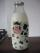Borrowed book

Jane must as soon as possible return a borrowed book. She figured that when she read 15 pages a day return book in time. Then she read 18 pages a day and then return the book one day before. How many pages should have a book?

Result

p =  90

Solution:

15d = p
18(d-1)=p

15d-p = 0
18d-p = 18

d = 6
p = 90

Calculated by our linear equations calculator.

Leave us a comment of this math problem and its solution (i.e. if it is still somewhat unclear...):Be the first to comment!To solve this verbal math problem are needed these knowledge from mathematics:

Do you have a linear equation or system of equations and looking for its solution? Or do you have quadratic equation?

Next similar math problems:

1. Every day 7 pagesAdelka reads the book every day 7 pages. When she reads one more page a day she will read it three days earlier. How long will Adelka read a book? How much does a book of pages have?
2. Unknown numberIdentify unknown number which 1/5 is 40 greater than one tenth of that number.
3. Forest nurseryIn the forest nursery after winter, they found that 1/10 stems died out of them. For them, they land 193 new spruces. How many spruces are in the forest nursery?
4. Equation?
5. Simple equationSolve the following simple equation: 2. (4x + 3) = 2-5. (1-x)
6. NormThree workers planted 3555 seedlings of tomatoes in one dey. First worked at the standard norm, the second planted 120 seedlings more and the third 135 seedlings more than the first worker. How many seedlings were standard norm?
7. Find xSolve: if 2(x-1)=14, then x= (solve an equation with one unknown)
8. Box of chocolatesIn a box of chocolates was 16 candies. Christopher and Luke they were distributed: a) Christopher had about 4 candies over Luke, b) Christopher had about 6 candies less than Luke, c) Christopher had 3 times more sweet than Luke. How many had each boy can
9. Simple equationSolve for x: 3(x + 2) = x - 18
10. Football match 4In a football match with the Italy lost 3 goals with Germans. Totally fell 5 goals in the match. Determine the number of goals of Italy and Germany.
11. EquationSolve the equation: 1/2-2/8 = 1/10; Write the result as a decimal number.
12. Tickets 3A total of 645 tickets were sold for the school play. They were either adult tickets or student tickets. There were 55 fewer student tickets sold than adult tickets. How many adult tickets were sold?
13. CakesGrandmother baked cakes. Half of its was poppy, quarter with plum jam and 16 cheesecakes. How many cakes she baked in total?
14. Football seasonThroughout the football season gave Adam the 23 goals more than Brano and 16 goals less than Karol. Edo gave the 6 goals more Dušan. Karol gave 5 more than Edo. Brano and Edo gave together 17 goals. How many goals gave each boy and how many goals gave the.
15. Boys and girlsThere are 48 children in the sports club, boys are 10 more than girls. How many girls go to the club?
16. TipplerBottle with cork cost 8.8 Eur. The bottle is 0.8 euros more expensive than cork. How much is a bottle and the cork?
17. Negative in equation2x + 3 + 7x = – 24, what is the value of x?